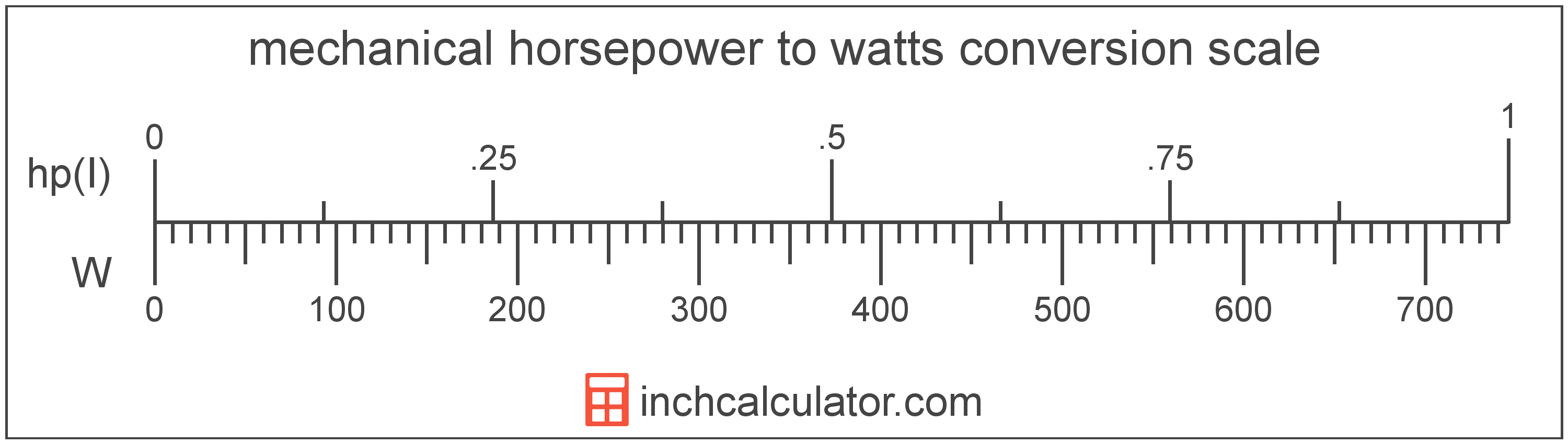# Convert Watts to Mechanical Horsepower

Enter the power in watts below to get the value converted to mechanical horsepower.

Results in Mechanical Horsepower:1 W = 0.001341 hp(I)

## How to Convert Watts to Mechanical HorsepowerTo convert a watt measurement to a mechanical horsepower measurement, multiply the power by the conversion ratio. One watt is equal to 0.001341 mechanical horsepower, so use this simple formula to convert:

mechanical horsepower = watts × 0.001341

The power in mechanical horsepower is equal to the watts multiplied by 0.001341.

For example, here's how to convert 5 watts to mechanical horsepower using the formula above.
5 W = (5 × 0.001341) = 0.006705 hp(I)

Watts and mechanical horsepower are both units used to measure power. Keep reading to learn more about each unit of measure.

## Watts

One watt is the power equal to the rate of energy of one joule per second in an electrical circuit. One watt is also equal to the power produced in a circuit with a voltage potential of one volt with a current of one amp.

The watt is the SI derived unit for power in the metric system. Watts can be abbreviated as W, for example 1 watt can be written as 1 W.

Watt's Law states the relationship between power, current, and voltage. Using Watt's Law, it's possible to express the power in watts as an expression using current and voltage.

PW = IA × VV

The power in watts is equal to the current in amperes times the potential difference in volts.

Combining this with Ohm's Law, it's also possible to express the power in watts using resistance in addition to volts and amps.

## Mechanical Horsepower

Mechanical horsepower is often used to measure the output of engines and motors in North America. One mechanical horsepower is equal to 745.69987158227022 watts.

The mechanical horsepower is a US customary and imperial unit of power. Mechanical horsepower can be abbreviated as hp(I), for example 1 mechanical horsepower can be written as 1 hp(I).

Try our engine horsepower calculator to calculate the power of a motor.

## Watt Measurements and Equivalent Mechanical Horsepower Conversions

Common watt values converted to the equivalent mechanical horsepower value
Watts Mechanical Horsepower
1 W 0.001341 hp(I)
2 W 0.002682 hp(I)
3 W 0.004023 hp(I)
4 W 0.005364 hp(I)
5 W 0.006705 hp(I)
6 W 0.008046 hp(I)
7 W 0.009387 hp(I)
8 W 0.010728 hp(I)
9 W 0.012069 hp(I)
10 W 0.01341 hp(I)
11 W 0.014751 hp(I)
12 W 0.016092 hp(I)
13 W 0.017433 hp(I)
14 W 0.018774 hp(I)
15 W 0.020115 hp(I)
16 W 0.021456 hp(I)
17 W 0.022797 hp(I)
18 W 0.024138 hp(I)
19 W 0.025479 hp(I)
20 W 0.02682 hp(I)
21 W 0.028161 hp(I)
22 W 0.029502 hp(I)
23 W 0.030844 hp(I)
24 W 0.032185 hp(I)
25 W 0.033526 hp(I)
26 W 0.034867 hp(I)
27 W 0.036208 hp(I)
28 W 0.037549 hp(I)
29 W 0.03889 hp(I)
30 W 0.040231 hp(I)
31 W 0.041572 hp(I)
32 W 0.042913 hp(I)
33 W 0.044254 hp(I)
34 W 0.045595 hp(I)
35 W 0.046936 hp(I)
36 W 0.048277 hp(I)
37 W 0.049618 hp(I)
38 W 0.050959 hp(I)
39 W 0.0523 hp(I)
40 W 0.053641 hp(I)

## More Watt Power Conversions

SI Units
Convert to Microwatts
1 W is equal to 1,000,000 microwatts
Convert to Milliwatts
1 W is equal to 1,000 milliwatts
Convert to Kilowatts
1 W is equal to 0.001 kilowatts
Convert to Megawatts
1 W is equal to 1.0E-6 megawatts
US Customary & Imperial Units
Convert to Electric Horsepower
1 W is equal to 0.00134 electric horsepower
Convert to Btu Per Hour
1 W is equal to 3.412142 btu per hour
Other Units
Convert to Kilocalories Per Hour
1 W is equal to 0.860421 kilocalories per hour